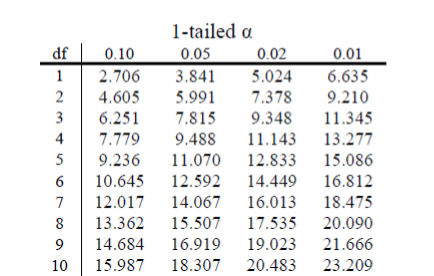# 14.2: Goodness-of-Fit

The first of our two $$\chi^{2}$$ tests assesses one categorical variable against a null hypothesis of equally sized frequencies. Equal frequency distributions are what we would expect to get if categorization was completely random. We could, in theory, also test against a specific distribution of category sizes if we have a good reason to (e.g. we have a solid foundation of how the regular population is distributed), but this is less common, so we will not deal with it in this text.

## Hypotheses

All $$\chi^{2}$$ tests, including the goodness-of-fit test, are non-parametric. This means that there is no population parameter we are estimating or testing against; we are working only with our sample data. Because of this, there are no mathematical statements for $$\chi^{2}$$ hypotheses. This should make sense because the mathematical hypothesis statements were always about population parameters (e.g. $$μ$$), so if we are non-parametric, we have no parameters and therefore no mathematical statements.

We do, however, still state our hypotheses verbally. For goodness-of-fit $$\chi^{2}$$ tests, our null hypothesis is that there is an equal number of observations in each category. That is, there is no difference between the categories in how prevalent they are. Our alternative hypothesis says that the categories do differ in their frequency. We do not have specific directions or one-tailed tests for $$\chi^{2}$$, matching our lack of mathematical statement.

## Degrees of Freedom and the $$\chi^{2}$$ table

Our degrees of freedom for the $$\chi^{2}$$ test are based on the number of categories we have in our variable, not on the number of people or observations like it was for our other tests. Luckily, they are still as simple to calculate:

$d f=C-1$

So for our pet preference example, we have 3 categories, so we have 2 degrees of freedom. Our degrees of freedom, along with our significance level (still defaulted to $$α = 0.05$$) are used to find our critical values in the $$\chi^{2}$$ table, which is shown in figure 1. Because we do not have directional hypotheses for $$\chi^{2}$$ tests, we do not need to differentiate between critical values for 1- or 2-tailed tests. In fact, just like our $$F$$ tests for regression and ANOVA, all $$\chi^{2}$$ tests are 1-tailed tests.Figure $$\PageIndex{1}$$: First 10 rows of the $$\chi^{2}$$ table

## Contributors

• Foster et al. (University of Missouri-St. Louis, Rice University, & University of Houston, Downtown Campus)